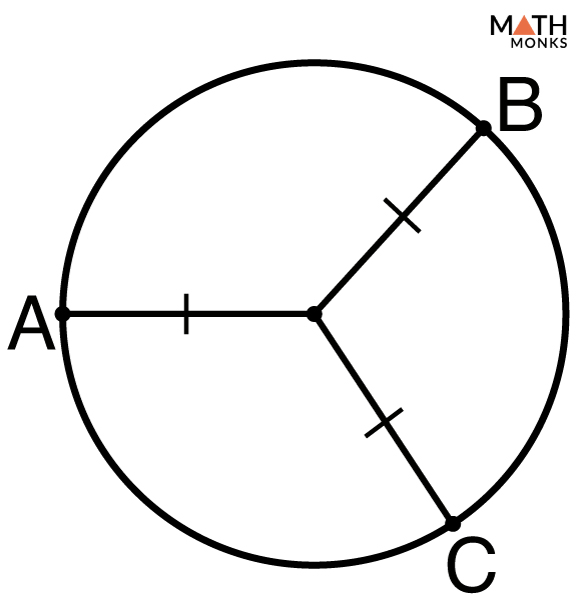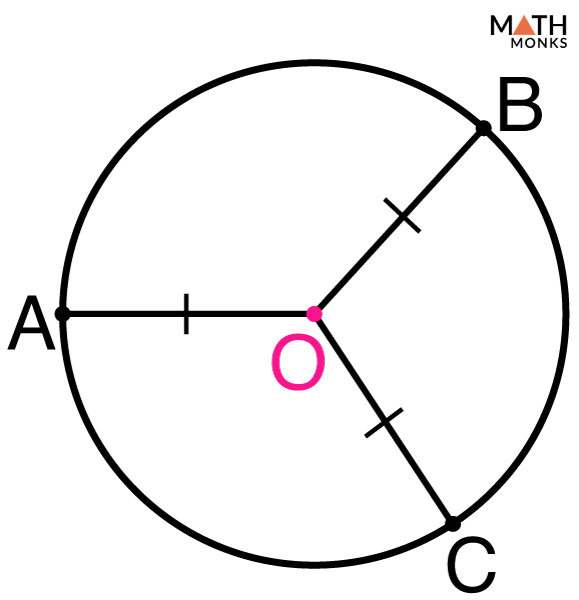# Center of a Circle

There are circles all around us in the real world such as a tire of a bicycle or a round wall clock. It is thus a familiar shape to us.

## What is the Center of a Circle

Center refers to the middle point of any object. According to the specific definition of a center, an object may not have a center.

The center of a circle is a point inside the circle that is equidistant from each point on the circumference of the circle. It is also called the focus of the circle. Shown below is the center of a circle ‘O’.

The center helps to form some of the other parts of the circle such as the radius or the diameter. The shortest distance from the center to any point on the circumference is called the radius. The diameter is the line segment connecting two points on the circumference of the circle and passing through the center. The radius is then used to find the area of the circumference of the circle.

## How to Find the Center of Circle

As discussed above, finding the center of a circle can help you perform basic geometric tasks like finding the circumference or area.

There are several ways to find the center. The 3 most widely used methods are shown below:

### Method 1: Drawing Crossed Lines

Steps

1. Draw a circle
2. Sketch a chord between two points
3. Draw a second chord
4. Make another line between A and C
5. Join B and D
6. Find the center

### Method 2: Using Overlapping Circles

Steps

1. Draw a chord between two points
2. Use a compass to draw two overlapping circles
3. Draw a vertical line through the two points at which the circles intersect
4. Erase the two overlapping circles
5. Sketch two new circles
6. Draw a line through the points at which these new circles intersect
7. Find the center

### Method 3: Using a Straightedge and a Triangular Ruler

Steps

1. Draw two straight, intersecting tangent lines onto the circle
2. Translate both of the lines to the other side of the circle
3. Draw the diagonals of the parallelogram
4. Check the accuracy of the center with a compass

## Solved ExamplesIdentify the center of the given circle.Solution:

In the given circle, ‘O’ is the center.

1.ilovetyping says: